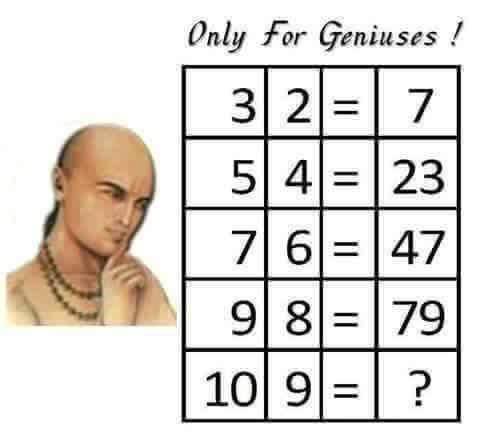# May 2016 Brain Teaser SolutionAnswer: 98
3 x 2 + 1 = 7
5 x 4 + 3 = 23
7 x 6 + 5 = 47
9 x 8 + 7 = 79
10 x 9 + 8 = 98